# Dealing with Rows and Columns in Pandas DataFrame

• Difficulty Level : Basic
• Last Updated : 13 Oct, 2021

A Data frame is a two-dimensional data structure, i.e., data is aligned in a tabular fashion in rows and columns. We can perform basic operations on rows/columns like selecting, deleting, adding, and renaming. In this article, we are using `nba.csv` file.### Dealing with Columns

In order to deal with columns, we perform basic operations on columns like selecting, deleting, adding and renaming.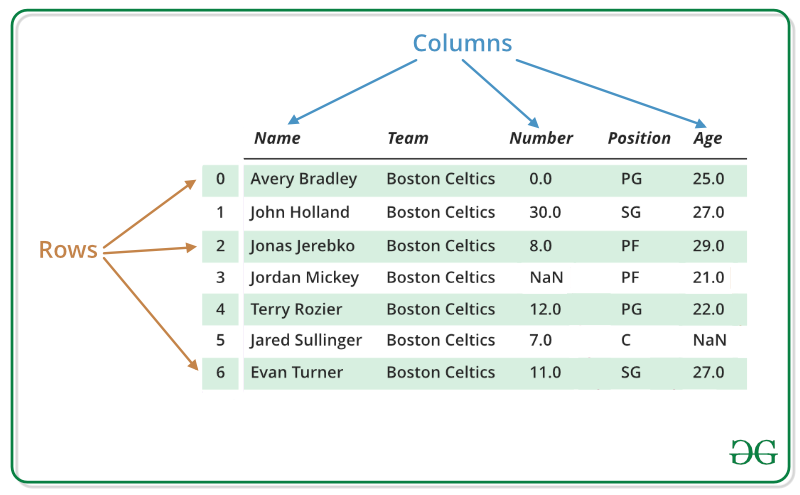Column Selection:
In Order to select a column in Pandas DataFrame, we can either access the columns by calling them by their columns name.

 `# Import pandas package ` `import` `pandas as pd ` ` `  `# Define a dictionary containing employee data ` `data ``=` `{``'Name'``:[``'Jai'``, ``'Princi'``, ``'Gaurav'``, ``'Anuj'``], ` `        ``'Age'``:[``27``, ``24``, ``22``, ``32``], ` `        ``'Address'``:[``'Delhi'``, ``'Kanpur'``, ``'Allahabad'``, ``'Kannauj'``], ` `        ``'Qualification'``:[``'Msc'``, ``'MA'``, ``'MCA'``, ``'Phd'``]} ` ` `  `# Convert the dictionary into DataFrame  ` `df ``=` `pd.DataFrame(data) ` ` `  `# select two columns ` `print``(df[[``'Name'``, ``'Qualification'``]]) `

Output: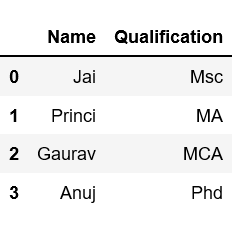For more examples refer to How to select multiple columns in a pandas dataframe

In Order to add a column in Pandas DataFrame, we can declare a new list as a column and add to a existing Dataframe.

 `# Import pandas package  ` `import` `pandas as pd ` ` `  `# Define a dictionary containing Students data ` `data ``=` `{``'Name'``: [``'Jai'``, ``'Princi'``, ``'Gaurav'``, ``'Anuj'``], ` `        ``'Height'``: [``5.1``, ``6.2``, ``5.1``, ``5.2``], ` `        ``'Qualification'``: [``'Msc'``, ``'MA'``, ``'Msc'``, ``'Msc'``]} ` ` `  `# Convert the dictionary into DataFrame ` `df ``=` `pd.DataFrame(data) ` ` `  `# Declare a list that is to be converted into a column ` `address ``=` `[``'Delhi'``, ``'Bangalore'``, ``'Chennai'``, ``'Patna'``] ` ` `  `# Using 'Address' as the column name ` `# and equating it to the list ` `df[``'Address'``] ``=` `address ` ` `  `# Observe the result ` `print``(df) `

Output: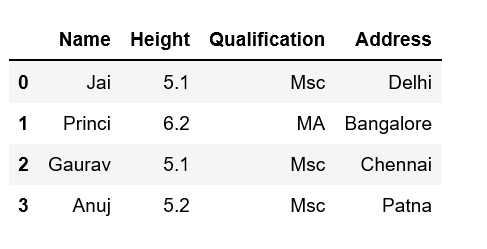For more examples refer to Adding new column to existing DataFrame in Pandas

Column Deletion:
In Order to delete a column in Pandas DataFrame, we can use the `drop()` method. Columns is deleted by dropping columns with column names.

 `# importing pandas module ` `import` `pandas as pd ` ` `  `# making data frame from csv file ` `data ``=` `pd.read_csv(``"nba.csv"``, index_col ``=``"Name"` `) ` ` `  `# dropping passed columns ` `data.drop([``"Team"``, ``"Weight"``], axis ``=` `1``, inplace ``=` `True``) ` ` `  `# display ` `print``(data) `

Output:
As shown in the output images, the new output doesn’t have the passed columns. Those values were dropped since axis was set equal to 1 and the changes were made in the original data frame since inplace was True.

Data Frame before Dropping Columns-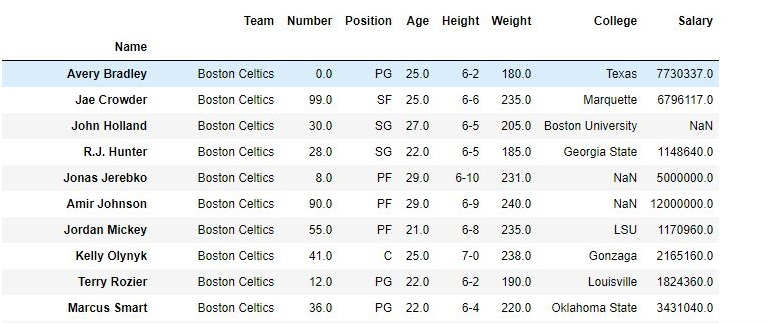Data Frame after Dropping Columns-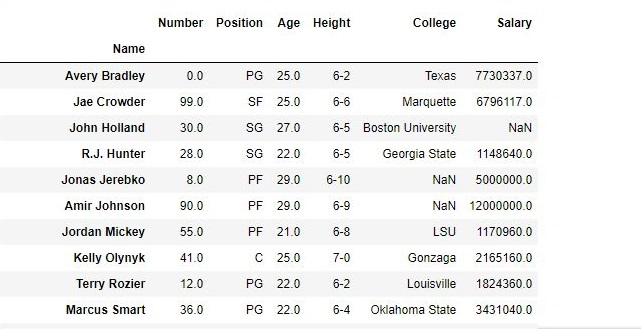For more examples refer to Delete columns from DataFrame using Pandas.drop()

### Dealing with Rows:

In order to deal with rows, we can perform basic operations on rows like selecting, deleting, adding and renaming.

Row Selection:
Pandas provide a unique method to retrieve rows from a Data frame.`DataFrame.loc[]` method is used to retrieve rows from Pandas DataFrame. Rows can also be selected by passing integer location to an iloc[] function.

 `# importing pandas package ` `import` `pandas as pd ` ` `  `# making data frame from csv file ` `data ``=` `pd.read_csv(``"nba.csv"``, index_col ``=``"Name"``) ` ` `  `# retrieving row by loc method ` `first ``=` `data.loc[``"Avery Bradley"``] ` `second ``=` `data.loc[``"R.J. Hunter"``] ` ` `  ` `  `print``(first, ``"\n\n\n"``, second) `

Output:
As shown in the output image, two series were returned since there was only one parameter both of the times.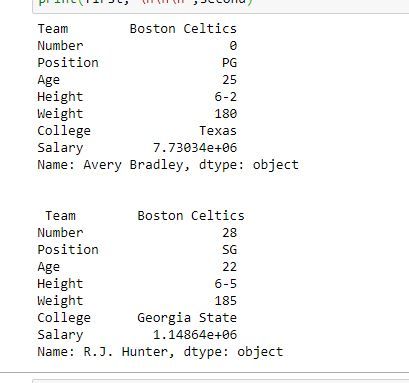For more examples refer to Pandas Extracting rows using .loc[]

In Order to add a Row in Pandas DataFrame, we can concat the old dataframe with new one.

 `# importing pandas module  ` `import` `pandas as pd  ` `   `  `# making data frame  ` `df ``=` `pd.read_csv(``"nba.csv"``, index_col ``=``"Name"``)  ` ` `  `df.head(``10``) ` ` `  `new_row ``=` `pd.DataFrame({``'Name'``:``'Geeks'``, ``'Team'``:``'Boston'``, ``'Number'``:``3``, ` `                        ``'Position'``:``'PG'``, ``'Age'``:``33``, ``'Height'``:``'6-2'``, ` `                        ``'Weight'``:``189``, ``'College'``:``'MIT'``, ``'Salary'``:``99999``}, ` `                                                            ``index ``=``[``0``]) ` `# simply concatenate both dataframes ` `df ``=` `pd.concat([new_row, df]).reset_index(drop ``=` `True``) ` `df.head(``5``) `

Output: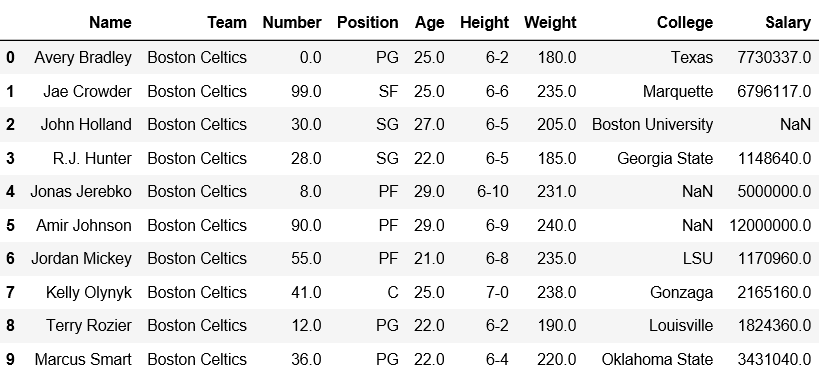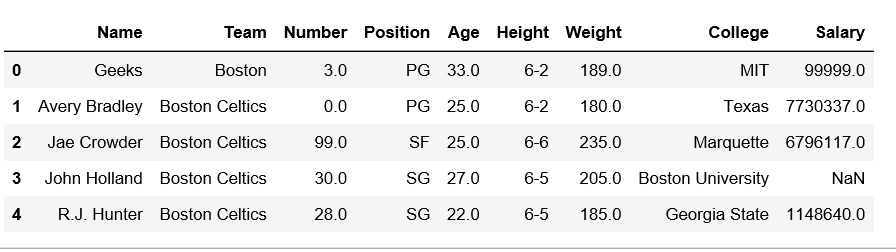For more examples refer to Add a row at top in pandas DataFrame

Row Deletion:
In Order to delete a row in Pandas DataFrame, we can use the drop() method. Rows is deleted by dropping Rows by index label.

 `# importing pandas module ` `import` `pandas as pd ` ` `  `# making data frame from csv file ` `data ``=` `pd.read_csv(``"nba.csv"``, index_col ``=``"Name"` `) ` ` `  `# dropping passed values ` `data.drop([``"Avery Bradley"``, ``"John Holland"``, ``"R.J. Hunter"``, ` `                            ``"R.J. Hunter"``], inplace ``=` `True``) ` ` `  `# display ` `data `

Output:
As shown in the output images, the new output doesn’t have the passed values. Those values were dropped and the changes were made in the original data frame since inplace was True.

Data Frame before Dropping values-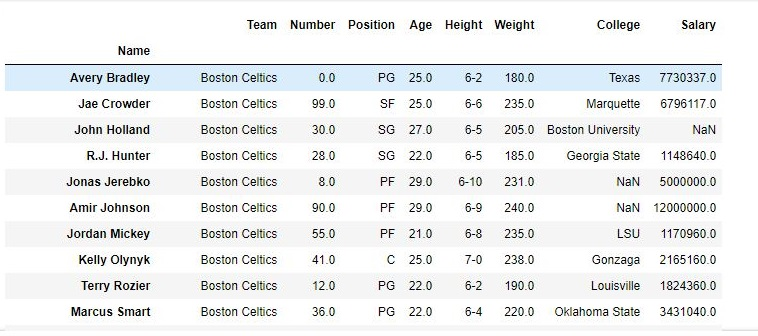Data Frame after Dropping values-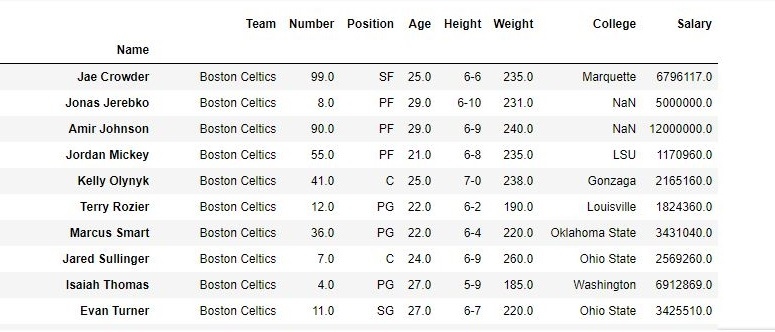For more examples refer to Delete rows from DataFrame using Pandas.drop()

Problem related to Columns:

Problem related to Rows:

My Personal Notes arrow_drop_up
Recommended Articles
Page :